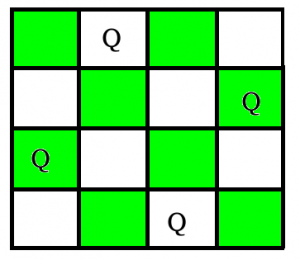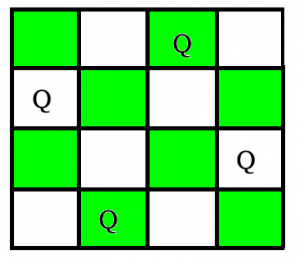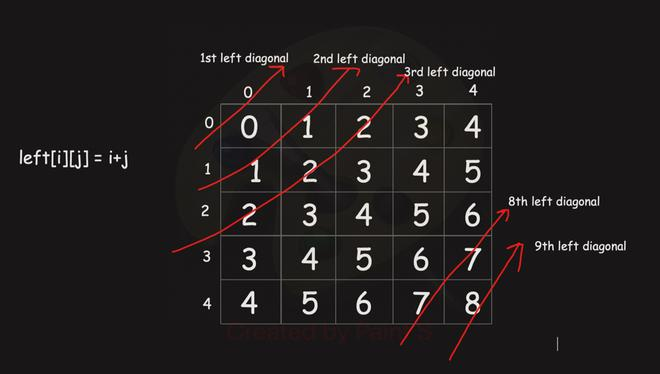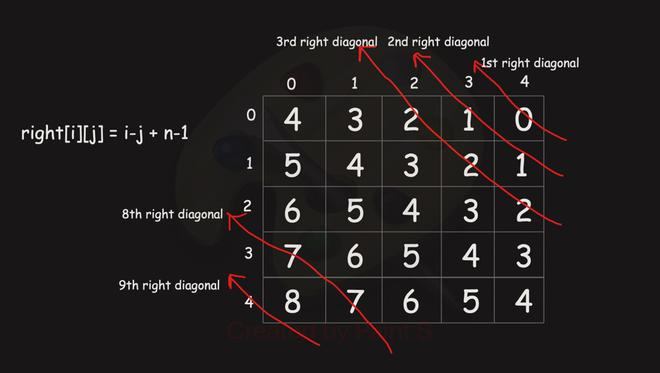# Printing all solutions in N-Queen Problem

• Difficulty Level : Hard
• Last Updated : 07 Oct, 2021

The N Queen is the problem of placing N chess queens on an N×N chessboard so that no two queens attack each other. For example, following is a solution for 4 Queen problem.
The N Queen is the problem of placing N chess queens on an N×N chessboard so that no two queens attack each other. For example, following are two solutions for 4 Queen problem.Attention reader! Don’t stop learning now. Get hold of all the important DSA concepts with the DSA Self Paced Course at a student-friendly price and become industry ready.  To complete your preparation from learning a language to DS Algo and many more,  please refer Complete Interview Preparation Course.

In case you wish to attend live classes with experts, please refer DSA Live Classes for Working Professionals and Competitive Programming Live for Students.In previous post, we have discussed an approach that prints only one possible solution, so now in this post, the task is to print all solutions in N-Queen Problem. Each solution contains distinct board configurations of the N-queens’ placement, where the solutions are a permutation of [1,2,3..n] in increasing order, here the number in the ith place denotes that the ith-column queen is placed in the row with that number. For the example above solution is written as [[2 4 1 3 ] [3 1 4 2 ]]. The solution discussed here is an extension of the same approach.

Backtracking Algorithm

The idea is to place queens one by one in different columns, starting from the leftmost column. When we place a queen in a column, we check for clashes with already placed queens. In the current column, if we find a row for which there is no clash, we mark this row and column as part of the solution. If we do not find such a row due to clashes then we backtrack and return false.

```1) Start in the leftmost column
2) If all queens are placed
return true
3) Try all rows in the current column.  Do following
for every tried row.
a) If the queen can be placed safely in this row
then mark this [row, column] as part of the
solution and recursively check if placing
queen here leads to a solution.
b) If placing queen in [row, column] leads to a
solution then return true.
c) If placing queen doesn't lead to a solution
then unmark this [row, column] (Backtrack)
and go to step (a) to try other rows.
3) If all rows have been tried and nothing worked,
return false to trigger backtracking.```

A modification is that we can find whether we have a previously placed queen in a column or in left diagonal or in right diagonal in O(1) time. We can observe that

1)For all cells in a particular left diagonal , their row + col  = constant.

2)For all cells in a particular right diagonal, their row – col + n – 1 = constant.

Let say n = 5, then we have a total of 2n-1 left and right diagonalsLet say we placed a queen at (2,0)

(2,0) have leftDiagonal value = 2. Now we can not place another queen at (1,1) and (0,2) because both of these have same leftDiagonal value as for (2,0). Similar thing can be noticed for right diagonal as well.

## C++

 `/* C/C++ program to solve N Queen Problem using``backtracking */``#include ``using` `namespace` `std;` `vector > result;` `/* A utility function to check if a queen can``be placed on board[row][col]. Note that this``function is called when "col" queens are``already placed in columns from 0 to col -1.``So we need to check only left side for``attacking queens */``bool` `isSafe(vector > board,``            ``int` `row, ``int` `col)``{``    ``int` `i, j;``    ``int` `N = board.size();` `    ``/* Check this row on left side */``    ``for` `(i = 0; i < col; i++)``        ``if` `(board[row][i])``            ``return` `false``;` `    ``/* Check upper diagonal on left side */``    ``for` `(i = row, j = col; i >= 0 && j >= 0; i--, j--)``        ``if` `(board[i][j])``            ``return` `false``;` `    ``/* Check lower diagonal on left side */``    ``for` `(i = row, j = col; j >= 0 && i < N; i++, j--)``        ``if` `(board[i][j])``            ``return` `false``;` `    ``return` `true``;``}` `/* A recursive utility function to solve N``Queen problem */``bool` `solveNQUtil(vector >& board, ``int` `col)``{``    ``/* base case: If all queens are placed``    ``then return true */``    ``int` `N = board.size();``    ``if` `(col == N) {``        ``vector<``int``> v;``        ``for` `(``int` `i = 0; i < N; i++) {``            ``for` `(``int` `j = 0; j < N; j++) {``                ``if` `(board[i][j] == 1)``                    ``v.push_back(j + 1);``            ``}``        ``}``        ``result.push_back(v);``        ``return` `true``;``    ``}` `    ``/* Consider this column and try placing``    ``this queen in all rows one by one */``    ``bool` `res = ``false``;``    ``for` `(``int` `i = 0; i < N; i++) {``        ``/* Check if queen can be placed on``        ``board[i][col] */``        ``if` `(isSafe(board, i, col)) {``            ``/* Place this queen in board[i][col] */``            ``board[i][col] = 1;` `            ``// Make result true if any placement``            ``// is possible``            ``res = solveNQUtil(board, col + 1) || res;` `            ``/* If placing queen in board[i][col]``            ``doesn't lead to a solution, then``            ``remove queen from board[i][col] */``            ``board[i][col] = 0; ``// BACKTRACK``        ``}``    ``}` `    ``/* If queen can not be place in any row in``        ``this column col then return false */``    ``return` `res;``}` `/* This function solves the N Queen problem using``Backtracking. It mainly uses solveNQUtil() to``solve the problem. It returns false if queens``cannot be placed, otherwise return true and``prints placement of queens in the form of 1s.``Please note that there may be more than one``solutions, this function prints one of the``feasible solutions.*/` `vector > nQueen(``int` `n)``{``    ``result.clear();``    ``vector > board(n, vector<``int``>(n, 0));` `    ``if` `(solveNQUtil(board, 0) == ``false``) {``        ``return` `{};``    ``}` `    ``sort(result.begin(), result.end());``    ``return` `result;``}` `// Driver Code``int` `main()``{``    ``int` `n = 4;``    ``vector > v = nQueen(n);` `    ``for` `(``auto` `ar : v) {``        ``cout << ``"["``;``        ``for` `(``auto` `it : ar)``            ``cout << it << ``" "``;``        ``cout << ``"]"``;``    ``}` `    ``return` `0;``}`

## Java

 `/* Java program to solve N Queen``Problem using backtracking */``import` `java.util.*;``class` `GfG {`` ` `  ``/* This function solves the N Queen problem using``    ``Backtracking. It mainly uses solveNQUtil() to``    ``solve the problem.``    ``*/``    ``static` `List> nQueen(``int` `n) {``       ``// cols[i] = true if there is a queen previously placed at ith column``        ``cols = ``new` `boolean``[n];``        ``// leftDiagonal[i] = true if there is a queen previously placed at``          ``// i = (row + col )th left diagonal``        ``leftDiagonal = ``new` `boolean``[``2``*n];``          ``// rightDiagonal[i] = true if there is a queen previously placed at``          ``// i = (row - col + n - 1)th rightDiagonal diagonal``        ``rightDiagonal = ``new` `boolean``[``2``*n];``        ``result  = ``new` `ArrayList<>();``        ``List temp = ``new` `ArrayList<>();``        ``for``(``int` `i=``0``;i> result,``int` `n,``int` `row,List comb){``        ``if``(row==n){``          ``// if row==n it means we have successfully placed all n queens.``          ``// hence add current arrangement to our answer``          ``// comb represent current combination``            ``result.add(``new` `ArrayList<>(comb));``            ``return``;``        ``}``        ``for``(``int` `col = ``0``;col > result``        ``= ``new` `ArrayList >();``   ``static` `boolean``[] cols,leftDiagonal,rightDiagonal;` `    ``// Driver code``    ``public` `static` `void` `main(String[] args)``    ``{``        ``int` `n = ``4``;` `        ``List > res = nQueen(n);``        ``System.out.println(res);``    ``}``}`

## Python3

 `''' Python3 program to solve N Queen Problem using``backtracking '''`  `result ``=` `[]` `# A utility function to print solution`  `''' A utility function to check if a queen can``be placed on board[row][col]. Note that this``function is called when "col" queens are``already placed in columns from 0 to col -1.``So we need to check only left side for``attacking queens '''`  `def` `isSafe(board, row, col):` `    ``# Check this row on left side``    ``for` `i ``in` `range``(col):``        ``if` `(board[row][i]):``            ``return` `False` `    ``# Check upper diagonal on left side``    ``i ``=` `row``    ``j ``=` `col``    ``while` `i >``=` `0` `and` `j >``=` `0``:``        ``if``(board[i][j]):``            ``return` `False``        ``i ``-``=` `1``        ``j ``-``=` `1` `    ``# Check lower diagonal on left side``    ``i ``=` `row``    ``j ``=` `col``    ``while` `j >``=` `0` `and` `i < ``4``:``        ``if``(board[i][j]):``            ``return` `False``        ``i ``=` `i ``+` `1``        ``j ``=` `j ``-` `1` `    ``return` `True`  `''' A recursive utility function to solve N``Queen problem '''`  `def` `solveNQUtil(board, col):``    ``''' base case: If all queens are placed``    ``then return true '''``    ``if` `(col ``=``=` `4``):``        ``v ``=` `[]``        ``for` `i ``in` `board:``          ``for` `j ``in` `range``(``len``(i)):``            ``if` `i[j] ``=``=` `1``:``              ``v.append(j``+``1``)``        ``result.append(v)``        ``return` `True` `    ``''' Consider this column and try placing``    ``this queen in all rows one by one '''``    ``res ``=` `False``    ``for` `i ``in` `range``(``4``):` `        ``''' Check if queen can be placed on``        ``board[i][col] '''``        ``if` `(isSafe(board, i, col)):` `            ``# Place this queen in board[i][col]``            ``board[i][col] ``=` `1` `            ``# Make result true if any placement``            ``# is possible``            ``res ``=` `solveNQUtil(board, col ``+` `1``) ``or` `res` `            ``''' If placing queen in board[i][col]``            ``doesn't lead to a solution, then``            ``remove queen from board[i][col] '''``            ``board[i][col] ``=` `0`  `# BACKTRACK` `    ``''' If queen can not be place in any row in``        ``this column col then return false '''``    ``return` `res`  `''' This function solves the N Queen problem using``Backtracking. It mainly uses solveNQUtil() to``solve the problem. It returns false if queens``cannot be placed, otherwise return true and``prints placement of queens in the form of 1s.``Please note that there may be more than one``solutions, this function prints one of the``feasible solutions.'''`  `def` `solveNQ(n):``    ``result.clear()``    ``board ``=` `[[``0` `for` `j ``in` `range``(n)]``             ``for` `i ``in` `range``(n)]``    ``solveNQUtil(board, ``0``)``    ``result.sort()``    ``return` `result`  `# Driver Code``n ``=` `4``res ``=` `solveNQ(n)``print``(res)` `# This code is contributed by YatinGupta`

## C#

 `/* C# program to solve N Queen``Problem using backtracking */``using` `System;``using` `System.Collections;``using` `System.Collections.Generic;` `class` `GfG {` `    ``static` `List > result = ``new` `List >();` `    ``/* A utility function to check if a queen can``    ``be placed on board[row,col]. Note that this``    ``function is called when "col" queens are``    ``already placed in columns from 0 to col -1.``    ``So we need to check only left side for``    ``attacking queens */``    ``static` `bool` `isSafe(``int``[, ] board, ``int` `row, ``int` `col,``                       ``int` `N)``    ``{``        ``int` `i, j;` `        ``/* Check this row on left side */``        ``for` `(i = 0; i < col; i++)``            ``if` `(board[row, i] == 1)``                ``return` `false``;` `        ``/* Check upper diagonal on left side */``        ``for` `(i = row, j = col; i >= 0 && j >= 0; i--, j--)``            ``if` `(board[i, j] == 1)``                ``return` `false``;` `        ``/* Check lower diagonal on left side */``        ``for` `(i = row, j = col; j >= 0 && i < N; i++, j--)``            ``if` `(board[i, j] == 1)``                ``return` `false``;` `        ``return` `true``;``    ``}` `    ``/* A recursive utility function``    ``to solve N Queen problem */``    ``static` `bool` `solveNQUtil(``int``[, ] board, ``int` `col, ``int` `N)``    ``{``        ``/* base case: If all queens are placed``        ``then return true */` `        ``if` `(col == N) {``            ``List<``int``> v = ``new` `List<``int``>();``            ``for` `(``int` `i = 0; i < N; i++)``                ``for` `(``int` `j = 0; j < N; j++) {``                    ``if` `(board[i, j] == 1)``                        ``v.Add(j + 1);``                ``}``            ``result.Add(v);``            ``return` `true``;``        ``}` `        ``/* Consider this column and try placing``        ``this queen in all rows one by one */``        ``bool` `res = ``false``;``        ``for` `(``int` `i = 0; i < N; i++) {``            ``/* Check if queen can be placed on``            ``board[i,col] */``            ``if` `(isSafe(board, i, col, N)) {``                ``/* Place this queen in board[i,col] */``                ``board[i, col] = 1;` `                ``// Make result true if any placement``                ``// is possible``                ``res = solveNQUtil(board, col + 1, N) || res;` `                ``/* If placing queen in board[i,col]``                ``doesn't lead to a solution, then``                ``remove queen from board[i,col] */``                ``board[i, col] = 0; ``// BACKTRACK``            ``}``        ``}` `        ``/* If queen can not be place in any row in``            ``this column col then return false */``        ``return` `res;``    ``}` `    ``/* This function solves the N Queen problem using``    ``Backtracking. It mainly uses solveNQUtil() to``    ``solve the problem. It returns false if queens``    ``cannot be placed, otherwise return true and``    ``prints placement of queens in the form of 1s.``    ``Please note that there may be more than one``    ``solutions, this function prints one of the``    ``feasible solutions.*/``    ``static` `List > solveNQ(``int` `n)``    ``{``        ``result.Clear();``        ``int``[, ] board = ``new` `int``[n, n];` `        ``solveNQUtil(board, 0, n);``        ``return` `result;``    ``}` `    ``// Driver code``    ``public` `static` `void` `Main()``    ``{``        ``int` `n = 4;``        ``List > res = solveNQ(n);``        ``for` `(``int` `i = 0; i < res.Count; i++) {``            ``Console.Write(``"["``);``            ``for` `(``int` `j = 0; j < res[i].Count; j++) {``                ``Console.Write(res[i][j]+ ``" "``);``            ``}``            ``Console.Write(``"]"``);``        ``}``    ``}``}` `/* This code contributed by PrinciRaj1992 */`
Output
`[2 4 1 3 ][3 1 4 2 ]`

Algorithm:
There is always only one queen in each row and each column, so idea of backtracking is to start placing queen from the leftmost column of each row and find a column where the queen could be placed without collision with previously placed queens. It is repeated from the first row till the last row. While placing a queen, it is tracked as if it is not making a collision (row-wise, column-wise and diagonally) with queens placed in previous rows. Once it is found that the queen can’t be placed at a particular column index in a row, the algorithm backtracks and change the position of the queen placed in the previous row then moves forward to place the queen in the next row.

1. Start with three-bit vector which is used to track safe place for queen placement row-wise, column-wise and diagonally in each iteration.
2. Three-bit vector will contain information as bellow:
• rowmask: set bit index (i) of this bit vector will indicate, the queen can’t be placed at ith column of next row.
• ldmask: set bit index (i) of this bit vector will indicate, the queen can’t e placed at ith column of next row. It represents the unsafe column index for next row falls under left diagonal of queens placed in previous rows.
• rdmask: set bit index (i) of this bit vector will indicate, the queen can’t be placed at ith column of next row. It represents the unsafe column index for next row falls right diagonal of queens placed in previous rows.
3. There is a 2-D (NxN) matrix (board), which will have ‘ ‘ character at all indexes in beginning and it gets filled by ‘Q’ row-by-row. Once all rows are filled by ‘Q’, the current solution is pushed into the result list.

Below is the implementation of the above approach:

## C++

 `// CPP program for above approach``#include ` `using` `namespace` `std;` `vector > result;``// Program to solve N Queens problem``void` `solveBoard(vector >& board, ``int` `row,``                ``int` `rowmask, ``int` `ldmask, ``int` `rdmask,``                ``int``& ways)``{` `    ``int` `n = board.size();` `    ``// All_rows_filled is a bit mask having all N bits set``    ``int` `all_rows_filled = (1 << n) - 1;` `    ``// If rowmask will have all bits set, means queen has``    ``// been placed successfully in all rows and board is``    ``// displayed``    ``if` `(rowmask == all_rows_filled) {` `        ``vector<``int``> v;``        ``for` `(``int` `i = 0; i < board.size(); i++) {``            ``for` `(``int` `j = 0; j < board.size(); j++) {``                ``if` `(board[i][j] == ``'Q'``)``                    ``v.push_back(j + 1);``            ``}``        ``}``        ``result.push_back(v);``        ``return``;``    ``}` `    ``// We extract a bit mask(safe) by rowmask,``    ``// ldmask and rdmask. all set bits of 'safe'``    ``// indicates the safe column index for queen``    ``// placement of this iteration for row index(row).``    ``int` `safe``        ``= all_rows_filled & (~(rowmask | ldmask | rdmask));``    ``while` `(safe) {` `        ``// Extracts the right-most set bit``        ``// (safe column index) where queen``        ``// can be placed for this row``        ``int` `p = safe & (-safe);``        ``int` `col = (``int``)log2(p);``        ``board[row][col] = ``'Q'``;` `        ``// This is very important:``        ``// we need to update rowmask, ldmask and rdmask``        ``// for next row as safe index for queen placement``        ``// will be decided by these three bit masks.` `        ``// We have all three rowmask, ldmask and``        ``// rdmask as 0 in beginning. Suppose, we are placing``        ``// queen at 1st column index at 0th row. rowmask,``        ``// ldmask and rdmask will change for next row as``        ``// below:` `        ``// rowmask's 1st bit will be set by OR operation``        ``// rowmask = 00000000000000000000000000000010` `        ``// ldmask will change by setting 1st``        ``// bit by OR operation  and left shifting``        ``// by 1 as it has to block the next column``        ``// of next row because that will fall on left``        ``// diagonal. ldmask =``        ``// 00000000000000000000000000000100` `        ``// rdmask will change by setting 1st bit``        ``// by OR operation and right shifting by 1``        ``// as it has to block the previous column``        ``// of next row because that will fall on right``        ``// diagonal. rdmask =``        ``// 00000000000000000000000000000001` `        ``// these bit masks will keep updated in each``        ``// iteration for next row``        ``solveBoard(board, row + 1, rowmask | p,``                   ``(ldmask | p) << 1, (rdmask | p) >> 1,``                   ``ways);` `        ``// Reset right-most set bit to 0 so,``        ``// next iteration will continue by placing the queen``        ``// at another safe column index of this row``        ``safe = safe & (safe - 1);` `        ``// Backtracking, replace 'Q' by ' '``        ``board[row][col] = ``' '``;``    ``}``    ``return``;``}` `// Driver Code``int` `main()``{``    ``// Board size``    ``int` `n = 4;``    ``int` `ways = 0;` `    ``vector > board;``    ``for` `(``int` `i = 0; i < n; i++) {``        ``vector<``char``> tmp;``        ``for` `(``int` `j = 0; j < n; j++) {``            ``tmp.push_back(``' '``);``        ``}``        ``board.push_back(tmp);``    ``}` `    ``int` `rowmask = 0, ldmask = 0, rdmask = 0;``    ``int` `row = 0;` `    ``// Function Call``    ``result.clear();``    ``solveBoard(board, row, rowmask, ldmask, rdmask, ways);``    ``sort(result.begin(),result.end());``    ``for` `(``auto` `ar : result) {``        ``cout << ``"["``;``        ``for` `(``auto` `it : ar)``            ``cout << it << ``" "``;``        ``cout << ``"]"``;``    ``}``    ``return` `0;``}``// This code is contributed by Nikhil Vinay`

## Java

 `// Java Program for above approach``import` `java.util.*;``public` `class` `NQueenSolution {` `    ``static` `List > result``        ``= ``new` `ArrayList >();` `    ``// Program to solve N-Queens Problem``    ``public` `void` `solveBoard(``char``[][] board, ``int` `row,``                           ``int` `rowmask, ``int` `ldmask,``                           ``int` `rdmask)``    ``{``        ``int` `n = board.length;` `        ``// All_rows_filled is a bit mask``        ``// having all N bits set``        ``int` `all_rows_filled = (``1` `<< n) - ``1``;` `        ``// If rowmask will have all bits set,``        ``// means queen has been placed successfully``        ``// in all rows and board is displayed``        ``if` `(rowmask == all_rows_filled) {``            ``List v = ``new` `ArrayList<>();` `            ``for` `(``int` `i = ``0``; i < n; i++) {``                ``for` `(``int` `j = ``0``; j < n; j++) {``                    ``if` `(board[i][j] == ``'Q'``)``                        ``v.add(j + ``1``);``                ``}``            ``}``            ``result.add(v);``            ``return``;``        ``}` `        ``// We extract a bit mask(safe) by rowmask,``        ``// ldmask and rdmask. all set bits of 'safe'``        ``// indicates the safe column index for queen``        ``// placement of this iteration for row index(row).``        ``int` `safe = all_rows_filled``                   ``& (~(rowmask | ldmask | rdmask));``        ``while` `(safe > ``0``) {` `            ``// Extracts the right-most set bit``            ``// (safe column index) where queen``            ``// can be placed for this row``            ``int` `p = safe & (-safe);``            ``int` `col = (``int``)(Math.log(p) / Math.log(``2``));``            ``board[row][col] = ``'Q'``;` `            ``// This is very important:``            ``// we need to update rowmask, ldmask and rdmask``            ``// for next row as safe index for queen``            ``// placement will be decided by these three bit``            ``// masks.` `            ``// We have all three rowmask, ldmask and``            ``// rdmask as 0 in beginning. Suppose, we are``            ``// placing queen at 1st column index at 0th row.``            ``// rowmask, ldmask and rdmask will change for``            ``// next row as below:` `            ``// rowmask's 1st bit will be set by OR operation``            ``// rowmask = 00000000000000000000000000000010` `            ``// ldmask will change by setting 1st``            ``// bit by OR operation  and left shifting``            ``// by 1 as it has to block the next column``            ``// of next row because that will fall on left``            ``// diagonal. ldmask =``            ``// 00000000000000000000000000000100` `            ``// rdmask will change by setting 1st bit``            ``// by OR operation and right shifting by 1``            ``// as it has to block the previous column``            ``// of next row because that will fall on right``            ``// diagonal. rdmask =``            ``// 00000000000000000000000000000001` `            ``// these bit masks will keep updated in each``            ``// iteration for next row``            ``solveBoard(board, row + ``1``, rowmask | p,``                       ``(ldmask | p) << ``1``,``                       ``(rdmask | p) >> ``1``);` `            ``// Reset right-most set bit to 0 so,``            ``// next iteration will continue by placing the``            ``// queen at another safe column index of this``            ``// row``            ``safe = safe & (safe - ``1``);` `            ``// Backtracking, replace 'Q' by ' '``            ``board[row][col] = ``' '``;``        ``}``    ``}` `    ``// Program to print board``    ``public` `void` `printBoard(``char``[][] board)``    ``{``        ``for` `(``int` `i = ``0``; i < board.length; i++) {``            ``System.out.print(``"|"``);``            ``for` `(``int` `j = ``0``; j < board[i].length; j++) {``                ``System.out.print(board[i][j] + ``"|"``);``            ``}``            ``System.out.println();``        ``}``    ``}` `    ``// Driver Code``    ``public` `static` `void` `main(String args[])``    ``{` `        ``// Board size``        ``int` `n = ``4``;` `        ``char` `board[][] = ``new` `char``[n][n];``        ``for` `(``int` `i = ``0``; i < n; i++) {``            ``for` `(``int` `j = ``0``; j < n; j++) {``                ``board[i][j] = ``' '``;``            ``}``        ``}` `        ``int` `rowmask = ``0``, ldmask = ``0``, rdmask = ``0``;``        ``int` `row = ``0``;` `        ``NQueenSolution solution = ``new` `NQueenSolution();` `        ``// Function Call``        ``result.clear();``        ``solution.solveBoard(board, row, rowmask, ldmask,``                            ``rdmask);``        ``System.out.println(result);``    ``}``}` `// This code is contributed by Nikhil Vinay`

## Python3

 `# Python program for above approach``import` `math` `result ``=` `[]` `# Program to solve N-Queens Problem`  `def` `solveBoard(board, row, rowmask,``               ``ldmask, rdmask):` `    ``n ``=` `len``(board)` `    ``# All_rows_filled is a bit mask``    ``# having all N bits set``    ``all_rows_filled ``=` `(``1` `<< n) ``-` `1` `    ``# If rowmask will have all bits set, means``    ``# queen has been placed successfully``    ``# in all rows and board is displayed``    ``if` `(rowmask ``=``=` `all_rows_filled):``        ``v ``=` `[]``        ``for` `i ``in` `board:``            ``for` `j ``in` `range``(``len``(i)):``                ``if` `i[j] ``=``=` `'Q'``:``                    ``v.append(j``+``1``)``        ``result.append(v)` `    ``# We extract a bit mask(safe) by rowmask,``    ``# ldmask and rdmask. all set bits of 'safe'``    ``# indicates the safe column index for queen``    ``# placement of this iteration for row index(row).``    ``safe ``=` `all_rows_filled & (~(rowmask |``                                ``ldmask | rdmask))` `    ``while` `(safe > ``0``):` `        ``# Extracts the right-most set bit``        ``# (safe column index) where queen``        ``# can be placed for this row``        ``p ``=` `safe & (``-``safe)``        ``col ``=` `(``int``)(math.log(p)``/``math.log(``2``))``        ``board[row][col] ``=` `'Q'` `        ``# This is very important:``        ``# we need to update rowmask, ldmask and rdmask``        ``# for next row as safe index for queen placement``        ``# will be decided by these three bit masks.` `        ``# We have all three rowmask, ldmask and``        ``# rdmask as 0 in beginning. Suppose, we are placing``        ``# queen at 1st column index at 0th row. rowmask, ldmask``        ``# and rdmask will change for next row as below:` `        ``# rowmask's 1st bit will be set by OR operation``        ``# rowmask = 00000000000000000000000000000010` `        ``# ldmask will change by setting 1st``        ``# bit by OR operation  and left shifting``        ``# by 1 as it has to block the next column``        ``# of next row because that will fall on left diagonal.``        ``# ldmask = 00000000000000000000000000000100` `        ``# rdmask will change by setting 1st bit``        ``# by OR operation and right shifting by 1``        ``# as it has to block the previous column``        ``# of next row because that will fall on right diagonal.``        ``# rdmask = 00000000000000000000000000000001` `        ``# these bit masks will keep updated in each``        ``# iteration for next row``        ``solveBoard(board, row``+``1``, rowmask | p,``                   ``(ldmask | p) << ``1``, (rdmask | p) >> ``1``)` `        ``# Reset right-most set bit to 0 so, next``        ``# iteration will continue by placing the queen``        ``# at another safe column index of this row``        ``safe ``=` `safe & (safe``-``1``)` `        ``# Backtracking, replace 'Q' by ' '``        ``board[row][col] ``=` `' '` `# Program to print board`  `def` `printBoard(board):``    ``for` `row ``in` `board:``        ``print``(``"|"` `+` `"|"``.join(row) ``+` `"|"``)` `# Driver Code`  `def` `main():` `    ``n ``=` `4`  `# board size``    ``board ``=` `[]` `    ``for` `i ``in` `range``(n):``        ``row ``=` `[]``        ``for` `j ``in` `range``(n):``            ``row.append(``' '``)``        ``board.append(row)` `    ``rowmask ``=` `0``    ``ldmask ``=` `0``    ``rdmask ``=` `0``    ``row ``=` `0` `    ``# Function Call``    ``result.clear()``    ``solveBoard(board, row, rowmask, ldmask, rdmask)``    ``result.sort()``    ``print``(result)`  `if` `__name__ ``=``=` `"__main__"``:``    ``main()` `# This code is contributed by Nikhil Vinay`
Output
`[2 4 1 3 ][3 1 4 2 ]`

This article is contributed by Sahil Chhabra. If you like GeeksforGeeks and would like to contribute, you can also write an article using write.geeksforgeeks.org or mail your article to review-team@geeksforgeeks.org. See your article appearing on the GeeksforGeeks main page and help other Geeks.